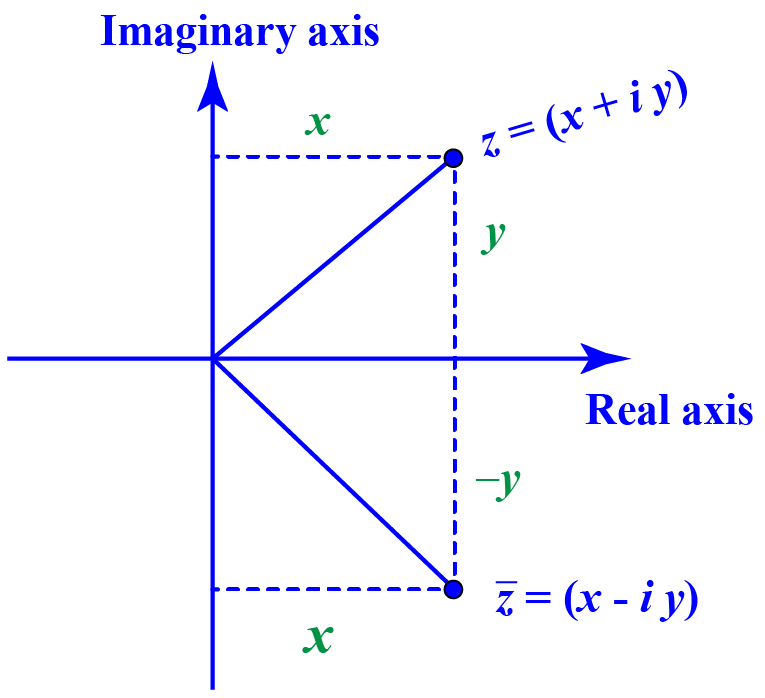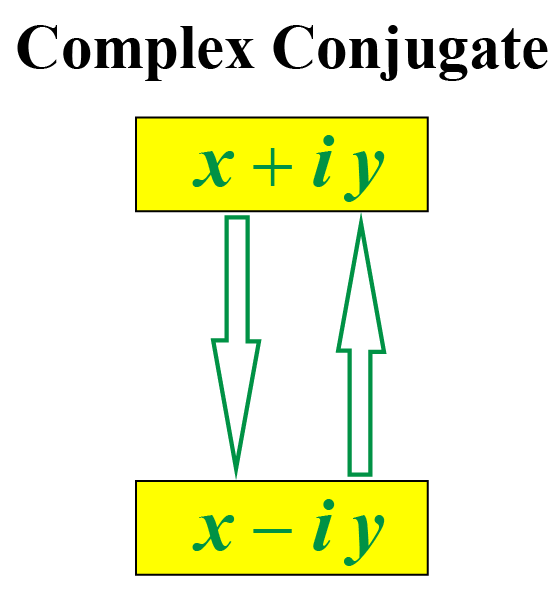# Complex Conjugate

We know that to add or subtract complex numbers, we just add or subtract their real and imaginary parts.

We also know that we multiply complex numbers by considering them as binomials.

But to divide two complex numbers, say $$\dfrac{1+i}{2-i}$$, we multiply and divide this fraction by $$2+i$$.

Here, $$2+i$$ is the complex conjugate of $$2-i$$.

Here is the complex conjugate calculator.

This will allow you to enter a complex number. Then it shows the complex conjugate of the complex number you have entered both algebraically and graphically.

Let's learn about complex conjugate in detail here.

## What Do You Mean by a Complex Conjugate?

### Definition of Complex Conjugate

The complex conjugate of a complex number, $$z$$, is its mirror image with respect to the horizontal axis (or x-axis).

The complex conjugate of $$z$$ is denoted by $$\bar{z}$$.Here $$z$$ and $$\bar{z}$$ are the complex conjugates of each other.

In the same way, if $$z$$ lies in quadrant II, can you think in which quadrant does $$\bar z$$ lie?

## How to Find the Conjugate of a Complex Number?

From the above figure, we can notice that the complex conjugate of a complex number is obtained by just changing the sign of the imaginary part.

• The complex conjugate of $$x+iy$$ is $$x-iy$$.
• The complex conjugate of $$x-iy$$ is $$x+iy$$.### Complex Conjugate Examples

Complex number
$$z$$

Its conjugate
$$\bar z$$

2 + 3i

2 - 3i

-1 - 5i

-1 + 5i
$$i-\dfrac{1}{2}$$ $$-i-\dfrac{1}{2}$$

## Properties of Complex Conjugates

We know that $$z$$ and $$\bar z$$ are conjugate pairs of complex numbers.

Here are the properties of complex conjugates.

• $$Re(z) = Re(\bar z)$$
• $$Im(z) =- Im(\bar z)$$
• If $$z$$ is purely real, then $$z=\bar z$$.
For example, the complex conjugate of $$z=3$$ is $$\bar z =3$$.
• If $$z$$ is purely imaginary, then $$z=-\bar z$$.
For example, the complex conjugate of $$z=3i$$ is $$\bar z = -3i$$.
• The bar over two complex numbers with some operation in between them can be distributed to each of the complex numbers. That is, if $$z_1$$ and $$z_2$$ are any two complex numbers, then:
\begin{align} \overline{z_1+z_2} &= \overline{z_1}+ \overline{z_2}\\[0.2cm] \overline{z_1-z_2}&= \overline{z_1}- \overline{z_2}\\[0.2cm] \overline{z_1 \cdot z_2}&= \overline{z_1} \cdot \overline{z_2}\\[0.2cm] \overline{\left(\ \dfrac{z_1}{z_2} \right)}&= \dfrac{ \overline{z_1}}{ \overline{z_2}}\end{align}Tips and Tricks
1. To divide two complex numbers, we multiply and divide with the complex conjugate of the denominator. For example:
$$\dfrac{1+i}{2-i} = \dfrac{1+i}{2-i} \cdot \color{blue}{\mathbf{\dfrac{2+i}{2+i}}}$$
2. We can use $$(x+iy)(x-iy) = x^2+y^2$$ when we multiply a complex number by its conjugate. This is because
\begin{align}&(x+iy)(x-iy)\0.2cm] &= (x)^2-(iy)^2 \\[0.2cm] &= x^2-i^2y^2\\[0.2cm] &= x^2+y^2\,\,\, \,\,\, [ \because i^2=-1] \end{align} Thus, the product of a complex number and its conjugate is always a positive real number. ## Solved Examples  Example 1 Can we help Emma find the complex conjugate of $$4 z_{1}-2 i z_{2}$$ given that $$z_{1}=2-3 i$$ and $$z_{2}=-4-7 i$$? Solution We will first find $$4 z_{1}-2 i z_{2}$$. \[ \begin{align} 4 z_{1}-2 i z_{2} &= 4(2-3i) -2i (-4-7i)\\[0.2cm] &= 8-12i+8i+14i^2\\[0.2cm] &= 8-12i+8i-14 \,\,\,[ \because i^2=-1]\\[0.2cm] &= -6 -4i \end{align}

The complex conjugate of $$4 z_{1}-2 i z_{2}= -6-4i$$ is obtained just by changing the sign of its imaginary part.

That is, $$\overline{4 z_{1}-2 i z_{2}}$$ is

 $$-6 + 4i$$
 Example 2

Can we help John find $$\dfrac{z_1}{z_2}$$ given that $$z_{1}=4-5 i$$ and $$z_{2}=-2+3 i$$?

Express the answer in the form of $$x+iy$$.

Solution

We have:

$\dfrac{z_{1}}{z_{2}}=\dfrac{4-5 i}{-2+3 i}$

To simplify this fraction, we have to multiply and divide this by the complex conjugate of the denominator, which is $$-2-3i$$.

\begin{align} \dfrac{z_{1}}{z_{2}}&=\dfrac{4-5 i}{-2+3 i} \times \dfrac{-2-3 i}{-2-3 i} \\[0.2cm] &=\dfrac{-8-12 i+10 i+15 i^{2}}{(-2)^{2}+(3)^{2}} \\[0.2cm] &=\dfrac{-8-12 i+10 i-15 }{(-2)^{2}+(3)^{2}}\,\,\, [ \because i^2=-1]\\[0.2cm] &=\dfrac{-23-2 i}{13}\\[0.2cm] &=-\dfrac{23}{13}+\left(-\dfrac{2}{13}\right) i \end{align}

Therefore,

 $$\dfrac{z_1}{z_2}=-\dfrac{23}{13}+\left(-\dfrac{2}{13}\right) i$$

## Interactive Questions

Here are a few activities for you to practice.

Select/type your answer and click the "Check Answer" button to see the result.

## Let's Summarize

The mini-lesson targeted the fascinating concept of Complex Conjugate. The math journey around Complex Conjugate starts with what a student already knows, and goes on to creatively crafting a fresh concept in the young minds. Done in a way that is not only relatable and easy to grasp but will also stay with them forever. Here lies the magic with Cuemath.

## About Cuemath

At Cuemath, our team of math experts is dedicated to making learning fun for our favorite readers, the students!

Through an interactive and engaging learning-teaching-learning approach, the teachers explore all angles of a topic.

Be it worksheets, online classes, doubt sessions, or any other form of relation, it’s the logical thinking and smart learning approach that we, at Cuemath, believe in.

## FAQs on Complex Conjugate

### 1. What is a complex conjugate?

The complex conjugate of a complex number is a complex number that can be obtained by changing the sign of the imaginary part of the given complex number.

For example, the complex conjugate of 2 + 3i is 2 - 3i.

### 2. What is the complex conjugate of 4i?

The complex conjugate of 4i is -4i.

### 3. What is the complex conjugate of z?

The complex conjugate of $$z$$ is denoted by $$\bar z$$ and is obtained by changing the sign of the imaginary part of $$z$$.

i.e., the complex conjugate of $$z=x+iy$$ is $$\bar z = x-iy$$ and vice versa.

### 4. What does conjugate mean?

The complex conjugate of a complex number, $$z$$, is its mirror image with respect to the horizontal axis (or x-axis).

### 5. What is a complex conjugate example?

Here are some complex conjugate examples:

Complex number
$$z$$

Its conjugate
$$\bar z$$

1 - 7i

1 + 7i

-2 - 5i

-2 + 5i
$$3i-\dfrac{3}{2}$$ $$-3i-\dfrac{3}{2}$$

### 6. What is the complex conjugate used for?

The complex conjugate is used to divide two complex numbers and get the result as a complex number.

### 7. What is the complex conjugate of i?

The complex conjugate of i is -i.

### 8. What are the properties of complex conjugates?

Here are the properties of complex conjugates.

• $$Re(z) = Re(\bar z)$$
• $$Im(z) =- Im(\bar z)$$
• If $$z$$ is purely real, then $$z=\bar z$$.
For example, the complex conjugate of $$z=3$$ is $$\bar z =3$$.
• If $$z$$ is purely imaginary, then $$z=-\bar z$$.
For example, the complex conjugate of $$z=2i$$ is $$\bar z = -3i$$.
• The bar over two complex numbers with some operation in between can be distributed to each of the complex numbers. i.e., if $$z_1$$ and $$z_2$$ are any two complex numbers, then:
\begin{align} \overline{z_1+z_2} &= \bar{z_1}+ \bar{z_2}\\[0.2cm] \overline{z_1-z_2}&= \bar{z_1}- \bar{z_2}\\[0.2cm] \overline{z_1 \cdot z_2}&= \bar{z_1} \cdot \bar{z_2}\\[0.2cm] \overline{\left(\ \dfrac{z_1}{z_2} \right)}&= \dfrac{ \bar{z_1}}{ \bar{z_2}}\end{align}
Download Complex Numbers Worksheets
Complex Numbers
grade 10 | Questions Set 1
Complex Numbers
grade 10 | Answers Set 1
Complex Numbers
grade 10 | Answers Set 2
Complex Numbers
grade 10 | Questions Set 2

More Important Topics
Numbers
Algebra
Geometry
Measurement
Money
Data
Trigonometry
Calculus